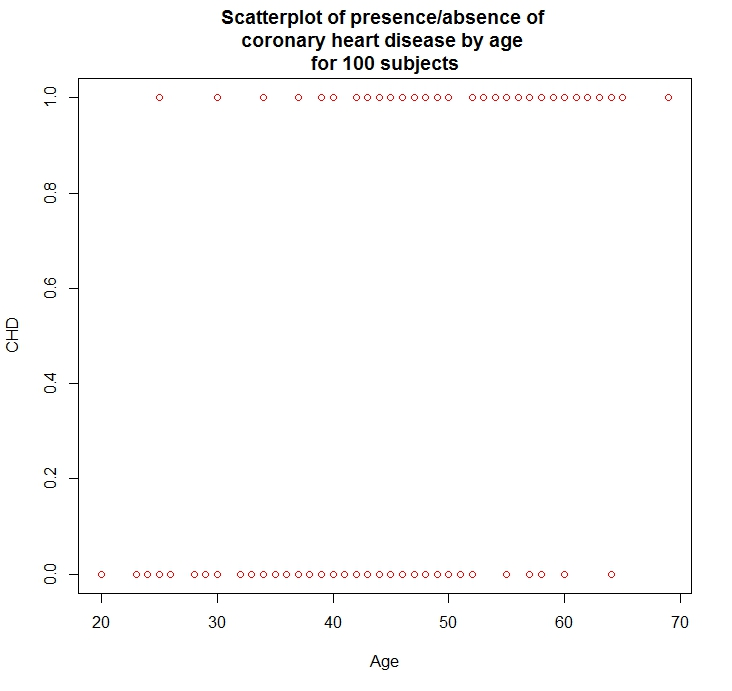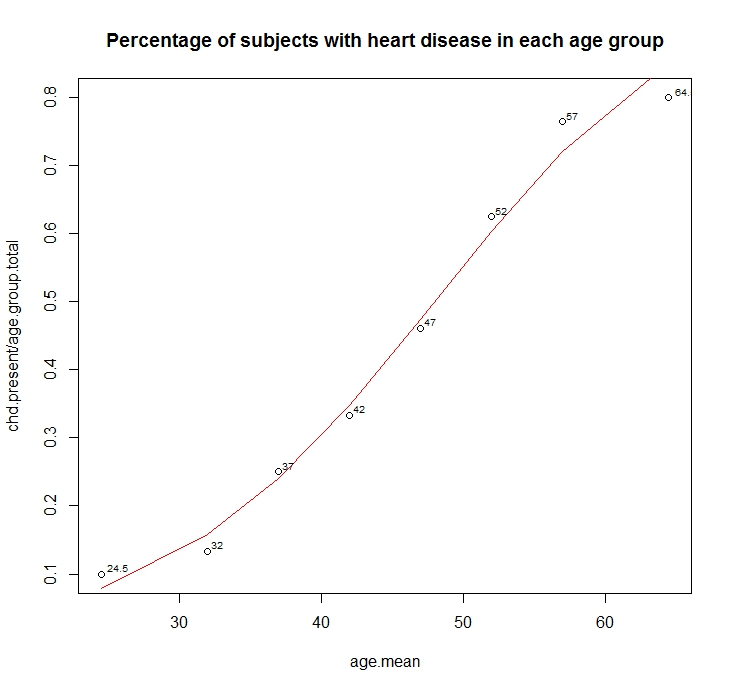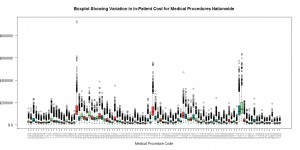# 06.23.13

## Heart-Disease Predictor Using Logistic Regression

Posted in Linear Regression at 10:48 am by Auro Tripathy

Probability is the very guide of life.
- Cicero

Given a two-column dataset, column one being age and column two being the presence/absence of heart-disease, we build a model (in R) that predicts the probability of heart-disease at an age. For a realistic model we aught to have big datasets with additional predictor variables such as blood-pressure, cholesterol, diabetes, smoking etc. However, the one-and-only predictor variable we have is age and the sample-size is 100 subjects!

Plotting the data (see below) doesn’t really provide a clear picture of the nature of the relationship between heart-disease and age. The problem is that the response variable (presence/absence of heart disease) is binary.Let’s create intervals of the independent variable (age) and compute the frequency of occurrence of the response variable (presence/absence of heart disease). You can get the table below  here.

A short and lucid tutorial in logistic regression is here (text) and here (video). The logistic curve is an S-shaped curve that takes the form,
y = [exp(b0 + b1x)] / [1 + exp(b0 + b1x)]

Clearly, the curve is non-linear, but the logit-transform makes it linear.
logit(y) = b0 + b1x

Thus, logistic regression is linear regression on the logit transform of y, where y is the probability of success at each value of x. Logistic regression fits b0 and b1, the regression coefficients.

The glm package in R is used to fit generalized regression models and can be used for logistic regression by specifying the family parameter to be binomial with the logit link like so:

```> glm.out = glm(cbind(chd.present, chd.absent) ~ age.mean,
+               family=binomial(logit), data=frequency.coronary.data)```

Plotting the fit shows us the close relationship between the fitted values and the observed values.Below is the R code that generated the plots.

```rm(list=ls())
plot(CHD ~ Age, data=coronary.data, col="red")
title(main="Scatterplot of presence/absence of \ncoronary heart disease by age \nfor 100 subjects")

library(calibrate) #needed to label observation
frequency.coronary.data[,"age.mean"] <- (frequency.coronary.data\$age.start +
frequency.coronary.data\$age.end)/2
frequency.coronary.data <- frequency.coronary.data[, c(1,2,6,3,4,5)] #reorder cols
#With "family=" set to "binomial" with a "logit" link,
# glm( ) produces a logistic regression
glm.fit = glm(cbind(chd.present, chd.absent) ~ age.mean,
family=binomial(logit), data=frequency.coronary.data)

summary(glm.fit)
plot(chd.present/age.group.total ~ age.mean, data=frequency.coronary.data)
lines(frequency.coronary.data\$age.mean, glm.fit\$fitted, type="l", col="red")

textxy(frequency.coronary.data\$age.mean,
frequency.coronary.data\$chd.present/frequency.coronary.data\$age.group.total,
frequency.coronary.data\$age.mean, cx=0.6)
title(main="Percentage of subjects with heart disease in each age group")```

Created by Pretty R at inside-R.org

References

2. http://ww2.coastal.edu/kingw/statistics/R-tutorials/logistic.html
3. Applied Logistic Regression, David W. Hosmer, Jr., Stanley Lemeshow, Rodney X. Sturdivant

# 06.17.13

## My First Brush w/Open Data – Hospital Charges

Posted in Data Visualization at 4:35 am by Auro Tripathy

Curious about what a medical procedure may cost you? Then, read on…

Recent data on the top 100 medical procedures is available here. The Government will soon release data on yet another 30 procedures. Below is a box plot showing the bewildering variation in in-patient cost for medical procedures nationwide.The R code below can be executed, without changes, to generate the plot above.

You can also use openrefine to discover that a medical procedure with code 207 can cost up to a million dollars!

```# Author Auro Tripathy, auro@shatterline.com

# Medicare Provider Charge Data: Inpatient
# The data provided here include hospital-specific charges for the more than 3,000 U.S. hospitals
# that receive Medicare Inpatient Prospective Payment System (IPPS) payments for the top 100 most
# frequently billed discharges, paid under Medicare based on a rate per discharge using the
# Medicare Severity Diagnosis Related Group (MS-DRG) for Fiscal Year (FY) 2011. These DRGs
# represent almost 7 million discharges or 60 percent of total Medicare IPPS discharges.

# http://www.cms.gov/Research-Statistics-Data-and-Systems/Statistics-Trends-and-Reports/Medicare-Provider-Charge-Data/Inpatient.html

rm(list=ls())
temp.zipped <- tempfile()
temp.zipped)
dim(hospital.charges)

#min/max needed to bound the plot
max <- max(hospital.charges\$Average.Covered.Charges)
min <- min(hospital.charges\$Average.Covered.Charges)

#if you want to study the data further, use openrefine (aka Google Refine)
colnames(hospital.charges)
unique(hospital.charges\$DRG.Definition)
unique(hospital.charges\$Provider.Zip.Code)
unique(hospital.charges\$Provider.Name)
unique(hospital.charges\$Provider.City)

procedures <- unique(hospital.charges\$DRG.Definition)

#procedure.by.charges.table
procedure.charges.array <- array(list(NULL), c(100))

for (i in 1:length(procedures)) {

procedure.charges <- hospital.charges[which(hospital.charges\$DRG.Definition == procedures[i]), ]
print(nrow(procedure.charges))

#used in the box-and-whiskers plot below
procedure.charges.array[[i]] <- array(procedure.charges\$Average.Covered.Charges, dim=nrow(procedure.charges))

}

#retain the three-digit medical code
procedures.labels <- as.character(procedures)
for (i in 1:length(procedures.labels)) {
procedures.labels[i] <- substr(procedures.labels[i], 1, 3)
}

#boxplot to show the media, the quartiles, and the outliers
boxplot(x = procedure.charges.array, main="Boxplot Showing Variation in In-Patient Cost for Medical Procedures Nationwide",
xlab="Medical Procedure Code",
col = c("lightgreen", "brown2", "cyan4"),
ylim=c(min, max), yaxt="n", col.ticks = "red", col.axis = "azure4", names=procedures.labels, las=2)

axis(2, axTicks(2), labels=sprintf("\$%2d", axTicks(2)), las=1)```

Created by Pretty R at inside-R.org